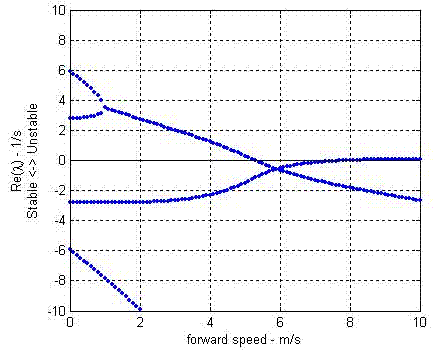# JBike6 Eigenvalues

#### EigenvaluesEigenvalues are defined as the scalar quantities λ such that for matrix [A], if there is a non-zero vector x for which this equation [A]x = λx is true, then x is an eigenvector of [A] and λ is its corresponding eigenvalue. Another way to think of them is that if the matrix [A] is a transformation, then the eigenvectors of [A] are those vectors whose directions are not changed by that transformation, and the corresponding eigenvalues are how much the magnitudes or lengths of those eigenvectors are changed.

You can read more at Numerical Computing with MATLAB at The MathWorks and MathWorld at Wolfram Research.

Here is yet a third way to think of eigenvalues. First, consider an eigenfunction, the essence of which is 'solutions which evolve to remain self-similar'. Anything which evolves in such a fashion only changes magnitude, in proportion to current magnitude, i.e. exponential behavior. In a sense the determination of eigenfunctions is the key question, and the resulting eigenvalue can then be determined by plugging in the eigenfunction and noting the magnitude change [after one multiplication of a transition matrix, which could be based on an elapsed time interval].

In fact, you may consider the classical approach, given initially above, starting with eigenvalues, to be backwards. It is merely the trick that most quickly leads to finding the eigenfunctions, or indeed the eigenvalues after recognizing that the eigenvalues alone are needed to answer a stability question.

For the upright bicycle, any perturbation motion consists of time evolving lean and steer, with initial conditions on lean amount, lean rate, steer amount, and steer rate. The whole theory of eigenfunctions is that there is a complete set of special 'fundamental' motions (which evolve in self-similar fashion), and since the system is linear, any disturbance can be decomposed into fundamental motions, and its whole future evolution easily calculated when they are added together.

Any linear time-evolving, time-independent equations have the property that the sum of two solutions is also a solution. Therefore there is special value in looking for 'eigensolutions' - solutions that remain self-similar as they evolve, and hence evolve exponentially. Then any solution can be expressed as linear combinations of eigensolutions. For the purpose of studying stability, only the exponential growth, defined by the 'eigenvalues', is really needed.

So, eigenvalues are often found by relatively simple calculations, on the way to determining eigensolutions. For example when written in state space form, eigenvalues they are identified as 'expansion numbers' that make it possible to find self-similar (exponential) solutions, by the vanishing of a determinant (polynomial).

Another excellent on-line article about eigenvalues, eigenvectors, eigenspaces, and some of their applications can be found in Wikipedia.